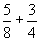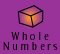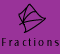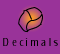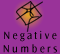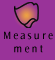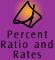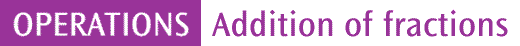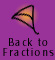| Steps for addition of fractions | Quick quiz |

We call fractions which have the same denominator like fractions. To add like fractions we just need to add the numerators.

Example 1: 1/4 + 3/4 = 1

 Question Setting Out Thinking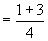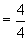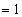If we add 3 quarters of a chocolate barto 1 quarter of a chocolate bar then we will get 4 quarters of a chocolate bar.4 quarters is equal to 1. So we have 1 chocolate bar.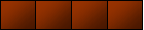Although we have shown the setting out above, we really don't need to do any working out to solve this problem. We can easily picture an object divided into 4 equal parts, quarters, and then add 3 quarters to 1 quarter.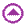Adding fractions with different denominators is not that much harder than adding like fractions. We just need to change the unlike fractions into like fractions.

Example 2: Adding unlike fractions using a model, 1/2 + 1/3 = 5/6

The model of the cake enabled us to picture this problem. We could also solve the problem without a model, as set out below:

 Question Setting Out Thinking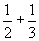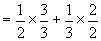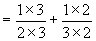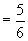If I want to add 1/2 and 1/3 I need to find a number which is a multiple of both 2 and 3, and make this the common denominator. 2 and 3 are factors of 6, so 6 will be a common denominator. So, I need to turn halves into sixths and thirds into sixths. I know a half is equivalent to 3 sixths so I can rename a half as 3 sixths. 1/2 = 3/6 (I could have changed one half into 3 sixths by multiplying by 3/3) I know one third is equivalent to 2 sixths so I can rename one third as two sixths. 1/3 = 2/6 (I could have changed 1 third into 2 sixths by multiplying by 2/2) Now I can add 3 sixths to 2 sixths to give 5 sixths.

Example 3: adding unlike fractions with unrelated denominators, 2/7 + 3/4 = 1 1/28

Example 4: adding unlike fractions with related denominators, 1/4 + 5/6 = 1 1/12

Example 5: adding unlike fractions with unrelated denominators, 3/4 + 4/5 = 1 11/20

Example 6: adding unlike fractions with related denominators, 2/3 + 7/15 = 1 2/15

Before you solve a mathematics problem it is important to have some idea about what the answer might be. Can you estimate the answer to this problem?

Example 7: 1 1/3 + 3 3/4 = ?

How did you estimate the answer? Did you:

• look for compatible numbers?
• group and add the whole numbers?
• recognise that the sum of 2 proper fractions will be less than 2?

We can use two methods to accurately find the answer to this question:

2. We can add the whole numbers first and then add the fractional parts.

We can change the mixed numbers into improper fractions and then add.

A full explanation of method 2 is shown below:
 Question Setting Out Thinking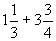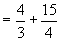To change 1 1/3 into 4/3 I need to change the '1' into thirds. 1 can be renamed as 3 thirds. Then I add the 1 third to give 4 thirds. 1 1/3 = 3/3 + 1/3 = 4/3 To change 3 3/4 into 15/4 I need to change the 3 into fourths (or quarters). 3 can be renamed as 12 quarters. Then I add the 3 quarters to give 15 quarters. 3 3/4 = 3 x 4/4 + 3/4 = 15/4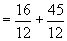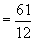In order to add thirds and fourths I need to find a common denominator. 3 and 4 are factors of 12, so 12 is a common denominator. 3 X 4 = 12 (denominator) 4/3 x 4/4 = 16/12 15/4 x 3/3 = 45/12 15 + 45 = 61 so 61 is the value of the numerator.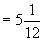5 x 12 = 60, so 60/12 = 5 and there is 1 left over which we write as 1/12. Is the answer close to our estimate?General rule for addition of fractions

There is a general rule which can be used for addition of fractions.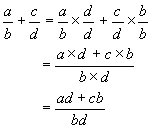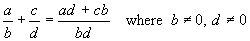However this method is not always the most efficient method to use to add fractions. Try using the rule to solve, 1/24 + 5/12 = ?

Example 8: Is there an easier way to find an answer to 1/24 + 5/12?

 To add fractions: Step 1: Check to see if the fractions have the same denominator. If they haven't then find a common denominator. Step 2: Make like fractions (i.e. with same denominator) Step 3: Add the like fractions Step 4: Simplify the answer if required.

Remember, if the question has mixed numbers, you can add the whole numbers first and then the fractions, or, you can change the mixed numbers to improper fractions and then add these fractions. In any case you must always find a common denominator before you add fractions.Quick quiz

 1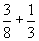2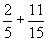3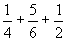4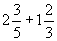56